# 比特币白皮书（中文版）

1． 介绍

2． 交易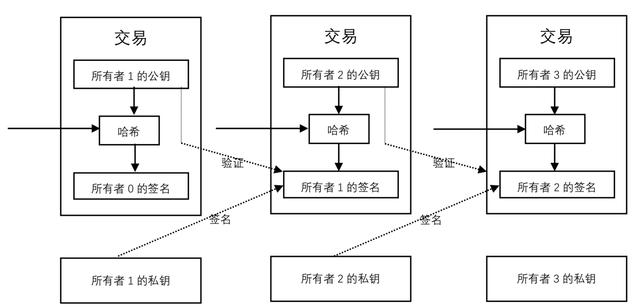3． 时间戳服务器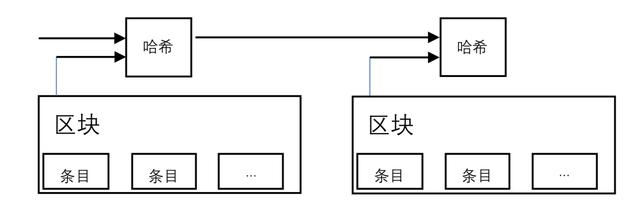4． 工作量证明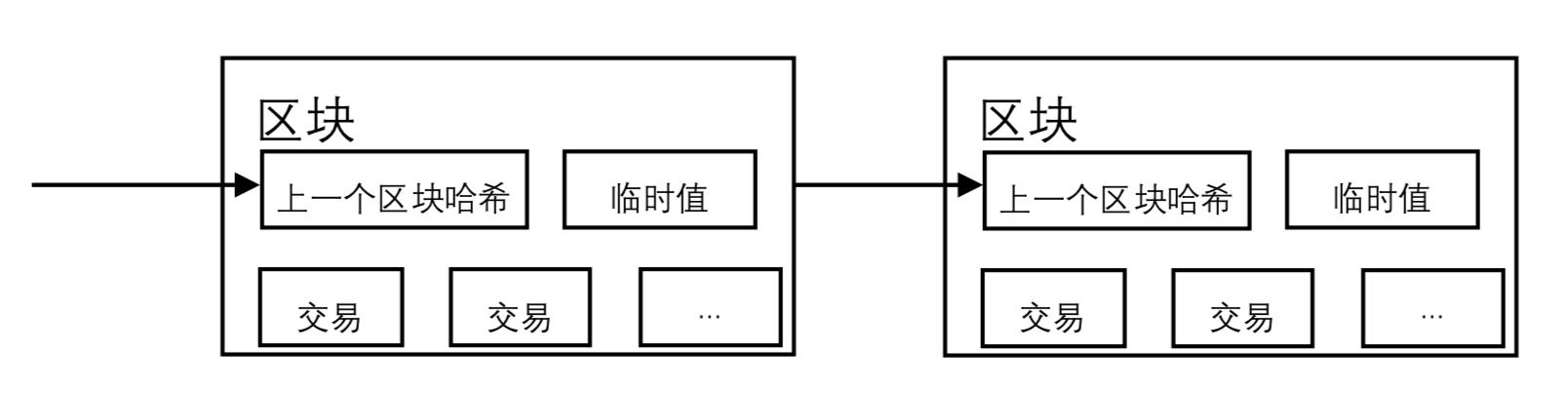5． 网络

1） 新交易给所有节点广播。

2） 每个节点将新交易放到一个区块。

3） 每个节点开始为这个区块寻找相应难度的工作量证明。

4） 当一个节点找到了这个工作量证明，把这个区块广播给所有节点。

5） 如果区块里所有的交易是有效的并且是没有被花费的，节点就会接受这个区块。

6） 节点把这个区块的哈希作为上一个哈希，并开始进行工作以竞争创建下一个区块。

6． 激励

7． 回收磁盘空间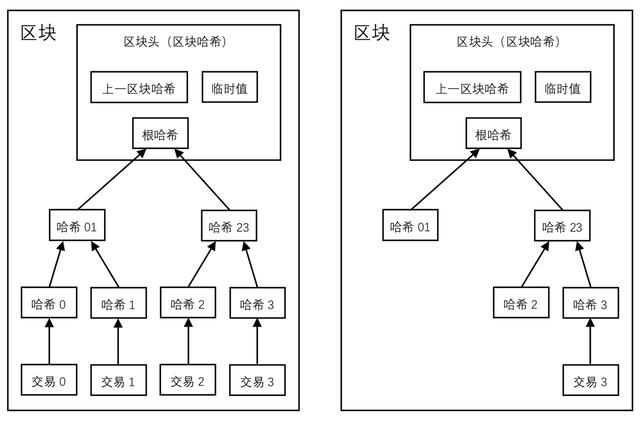8． 简化支付验证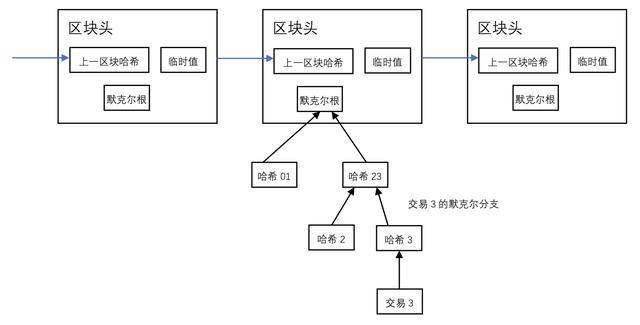9． 组合和分割价值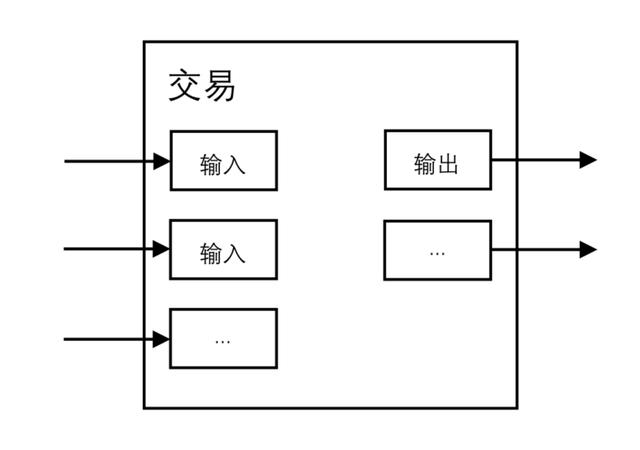10． 隐私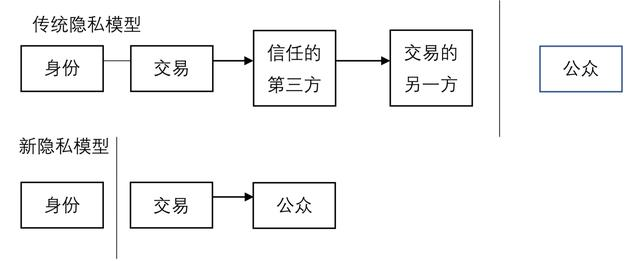11． 计算

p=诚实节点发现下一个区块的概率

q=攻击者找到下一个区块的概率

qz =攻击者在落后z个区块的情况下，追上的概率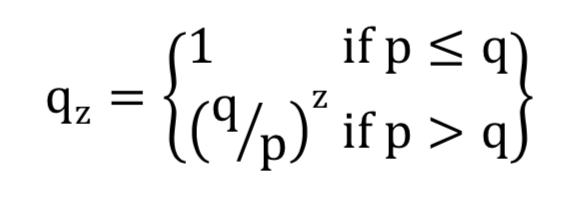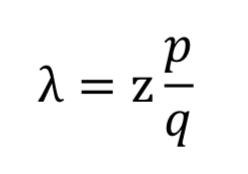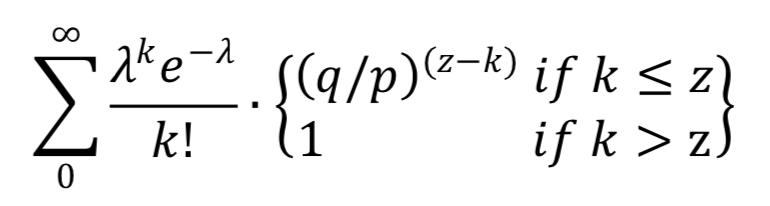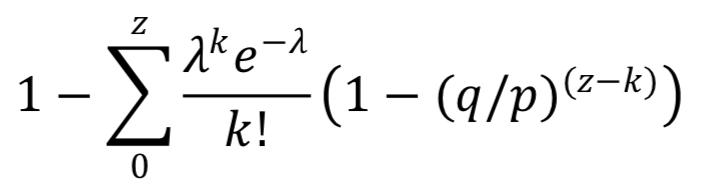#include <math.h>

double AttackerSuccessProbability(double q, int z)

{

double p = 1.0 - q;

double lambda = z * (q / p);

double sum = 1.0;

int i, k;

for (k = 0; k <= z; k++)

{

double poisson = exp(-lambda);

for (i = 1; i <= k; i++)

poisson *= lambda / i;

sum -= poisson * (1 - pow(q / p, z - k));

}

return sum;

}

q=0.1

z=0 P=1.0000000

z=1 P=0.2045873

z=2 P=0.0509779

z=3 P=0.0131722

z=4 P=0.0034552

z=5 P=0.0009137

z=6 P=0.0002428

z=7 P=0.0000647

z=8 P=0.0000173

z=9 P=0.0000046

z=10 P=0.0000012

q=0.3

z=0 P=1.0000000

z=5 P=0.1773523

z=10 P=0.0416605

z=15 P=0.0101008

z=20 P=0.0024804

z=25 P=0.0006132

z=30 P=0.0001522

z=35 P=0.0000379

z=40 P=0.0000095

z=45 P=0.0000024

z=50 P=0.0000006

p < 0.001

q=0.10 z=5

q=0.15 z=8

q=0.20 z=11

q=0.25 z=15

q=0.30 z=24

q=0.35 z=41

q=0.40 z=89

q=0.45 z=340

12． 结论

08-0310-2922万+
06-113318
06-124321
12-16119
07-20
12-19
11-05
07-07865
02-091万+
03-07979
02-21
03-21
09-22
©️2020 CSDN 皮肤主题: 大白 设计师:CSDN官方博客